Fatigue Index

Fatigue Action Types

Fatigue Action types

The fatigue action types refers to the types of stress loading that results in fatigue failures in engineering materials.

Alternating /Reversing Stress

In determining fatigue stress levels using standard test equipment the test specimens are subject to alternative/reversed stress levels... The cyclic stress varies from σ a tensile to σ a compressive.   The mean stress = 0.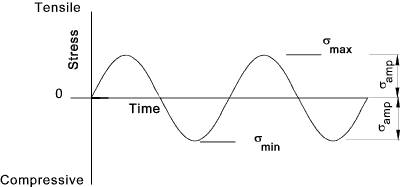The fatigue strength values for metals are based on this loading condition with the published fatigue strength values S'n, representing the material fatigue strength property, being the σ amp value at failure.

Repeated Stress

In the repeated stress loading condition the stress varies between zero and a maximum tensile (or compressive) stress in a cyclic manner...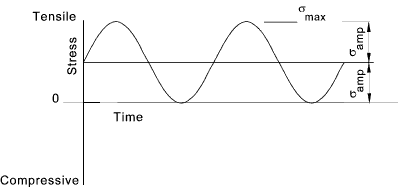A stress condition normally experienced in practice is a cyclic stress imposed on a steady load stress..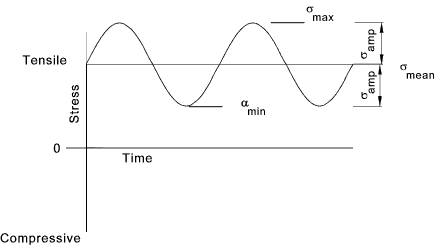The maximum stress = σmax The minimum stress = σmin The mean stress = σmean = ( σmax + σmin ) / 2 The stress Amplitude= σamp = ( σmax - σmin ) / 2 The stress Ratio R = σmin / σmax

For Alternating Stress... R = -1
For Repeated Stress +ve... R = 0 / σ max
For Repeated Stress -ve... R = σ min / 0

Combination of Mean Stress and Fluctuating stress

The mean stress can have a significant effect on the failure due to fatigue and must be considered in combination with the alternating stress..(Under normal fatigue loading conditions the mean stress is small compared to the alternating stress.)

A number of interaction criteria are used to quantify the combined stress and the relevant design factors of safety..These are plotted together below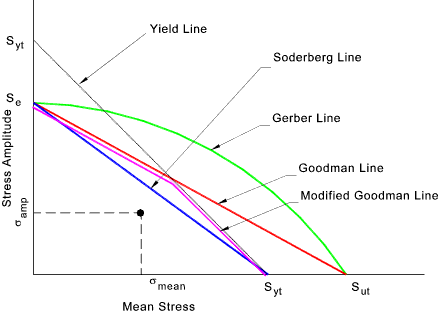The Mean Stress σ mean is plotted on the horizontal axis and the alternating stress σampis plotted on the vertical axis.

Soderberg Line

If the point of the combined stress is below the Soderberg line then the component will not fail . This is a very conservative criteria based on the material yield point Syt
To establish the factor of safety relative to the Soderberg Criteria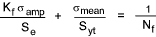Goodman Line /Modified Goodman Line

If the point of the combined stress is below the relevant Goodman line then the component will not fail . This is a less conservative criteria based on the material Ultimate strength ield point Sut
To establish the factor of safety relative to the Goodman Criteria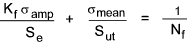Gerber Line

If the point of the combined stress is below the Gerber line then the component will not fail . This is a less conservative criteria based on the material Ultimate strength Sut
To establish the factor of safety relative to the Gerber Criteria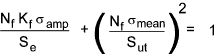Se = The Modified fatigue strength Sut = The ultimate tensile strength Syt = The yield tensile strength Nf = The Factor of Safety applicable the Fatigue

 Useful Links.. Efatigue.com... Excellent Source of Fatigue data. (Based on US materials )
Fatigue Index